cancel
Showing results for
Did you mean:## Decoding Direct Query in Power BI Part 1: Time Intelligence in Direct Query : SQL Server

The time Intelligence function remain the favorite of the Power BI developer, as they allow easy calculation for MTD, QTD, YTD and prior period for the same. But how to achieve the same in a direct query environment?

The questions we are going to address.

1. How to create a Date table in case of Direct Query
2. How to change the Data type in case of Direct Query
3. How to attach a sort column
4. How to use time intelligence functions

For this blog article, we have used the SQL Server 17 Express edition.  And the latest version of Power BI Desktop with the new Office Ribbon enabled. In my previous blog, I have used the sales data set. We are going to use the same dataset here with a few modifications.

As SQL Server Express does not allow to load data from Excel, we converted all data to CSV files and loaded them to the SQL Server Express edition. We also created a Date table. With Date, Month-Year, Month-Year Sort and Year(Numeric).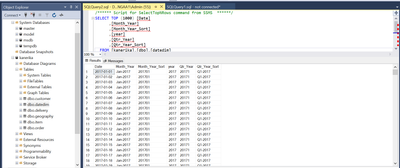Step 1:

Connected SQL server, and while connecting choose Direct Query Option.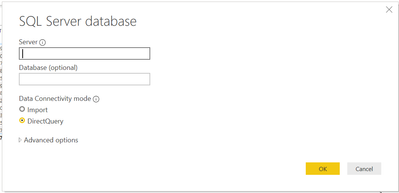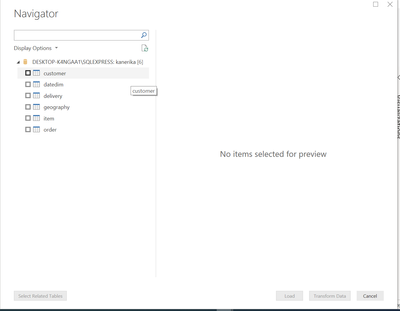Step 2:

Drag all the required tables into Power BI and create the relationship diagram, as given below. Take two facts and you can see both are connected to the common dimensions. In this example, we are going to use only one.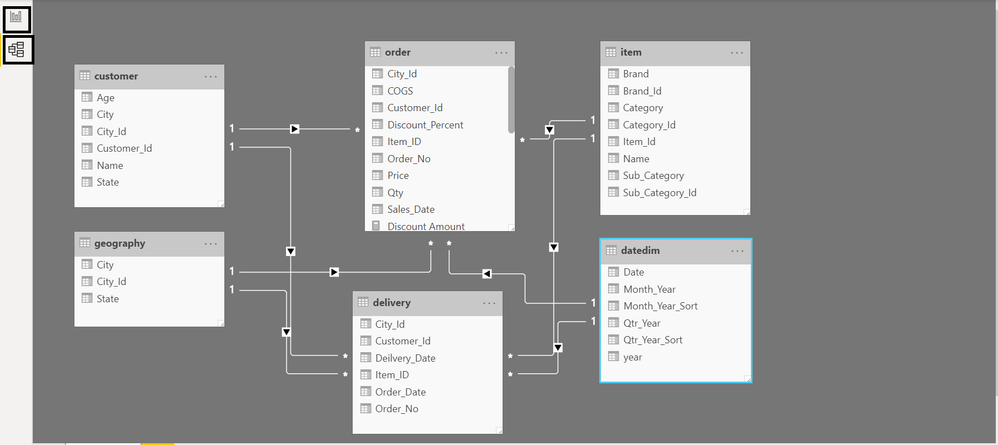Step 3: Mark Time Table

Where is the option? We do not have the Data view. Just right click on the table in the Visualization tab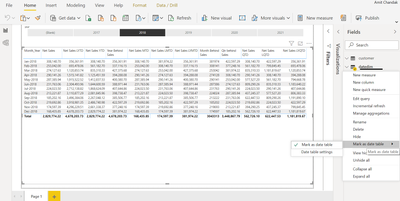Step 4: Change Data Type

Click on any table field/column. You will see column tools as one of the tabs. You have the option to change the data type there. I wanted to make year text in the date dimension.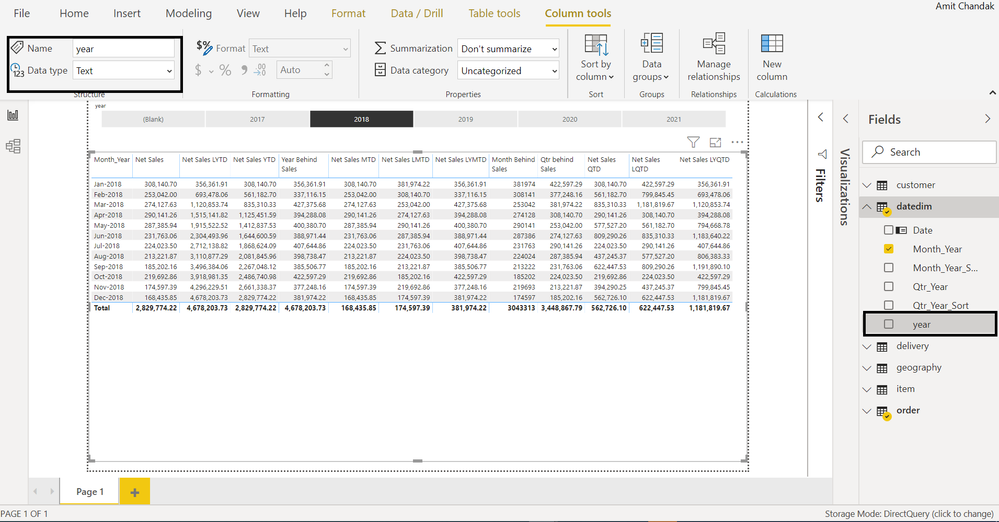Step 5: Change Sort column

Again, under Column Tools, you have the option for Sort by column. We used that. We have changed sort for Month Year.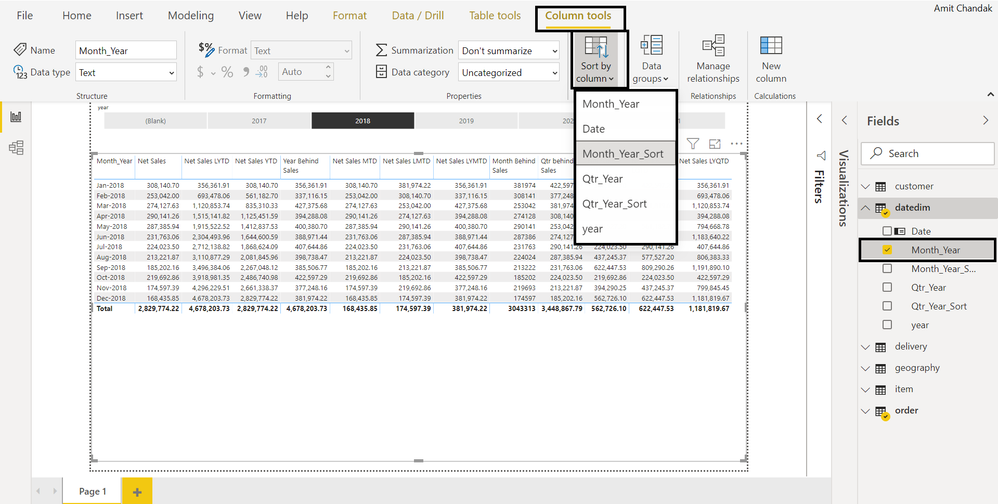Step 6: Create formulas

Our data do not have Gross Sales or Net Sales as values. So, we needed new measures for those. Note that these calculations are needed at the line level. So, we used sumx function, as we do not have the option to create a column. Refer to the last blog to see how they were calculated as columns.

``````Net Sales = sumx('order',('order'[Qty]*'order'[Price])* (100-'order'[Discount_Percent])/100)
Gross Sales = sumx('order','order'[Qty]*'order'[Price])
Discount Amount = sumx('order',('order'[Qty]*'order'[Price])*'order'[Discount_Percent]/100)``````Step 7: Create the Time Intelligence formula

We have created formulas using dates functions like datesytd, datesqtd and datesmtd. We have also created trailing/behind a month, quarter and year measures. They worked as expected.

``````Net Sales MTD = CALCULATE([Net Sales],DATESMTD(datedim[Date]))
Net Sales QTD = CALCULATE([Net Sales],DATESQTD(datedim[Date]))
Net Sales YTD = CALCULATE([Net Sales],DATESYTD(datedim[Date]))

Net Sales LMTD = CALCULATE([Net Sales],DATESMTD(DATEADD(datedim[Date],-1,MONTH)) )
Net Sales LQTD = CALCULATE([Net Sales],DATESQTD(DATEADD(datedim[Date],-1,QUARTER)))

Net Sales LYMTD = CALCULATE([Net Sales],DATESMTD(DATEADD(datedim[Date],-1,YEAR)) )
Net Sales LYQTD = CALCULATE([Net Sales],DATESQTD(DATEADD(datedim[Date],-1,YEAR)) )
Net Sales LYTD = CALCULATE([Net Sales],DATESYTD(DATEADD(datedim[Date],-1,YEAR)) )

Month Behind Sales = CALCULATE([Net Sales],DATEADD(datedim[Date],-1,MONTH))
Qtr behind Sales = CALCULATE([Net Sales],DATEADD(datedim[Date],-1,QUARTER))
Year Behind Sales = CALCULATE([Net Sales],DATEADD(datedim[Date],-1,year))``````These are not different from what we use in the import query mode.

Do you want us to cover any other topic on Direct Query Mode? Comment and let us know.

This time, I'm not attaching the pbix, as you might have Database access and it will not work.